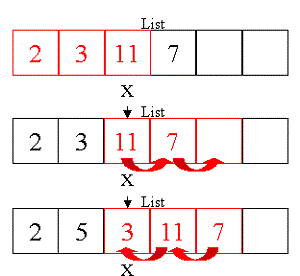# Java ArraysJava arrays allow use to store multiple values of the same type. Array elements are accessible by their indices. 0 is the first index. arrayname.length gives the length of the array. See example below. First, an int array of 5 elements is declared. Then values 1 and 2 are assigned to the first two elements. Note the use of indices 0 and 1. Next the value of the second element is printed. Loops are used to access multiple values in an array. The for loop prints the values of all elements. Arrays are initialized with default values, which for int is 0. Thus the result 1 2 0 0 0. The second array is a String array. A size is not defined since it is initialized upon declaration and the size is the number of initialized elements. The following line of code modifies the value of an existing element. Finally the for loop prints all the values in the array. Note that b.length is used instead of 4.

``````public class ArrayExp {
public static void main(String[] args) {
// declare an array of 5 elements
int[] a = new int;

// assign values to elements
a = 1;
a = 2;

// printing value of an element
System.out.println(a);

// loop through the entire array
for (int i = 0; i &lt; 5; i++) {
System.out.print(a[i] + " ");
// output: 1 2 0 0 0
// note empty elements are set to 0
}
System.out.println();

// declare a String array.
// initialized upon declaration
String[] b = {"an","in","am","no"};

// modifying value of an element
b = "out";

// note the use of b.length
for (int i = 0; i < b.length; i++) {
System.out.print(b[i] + " ");
}
System.out.println();
}
}``````